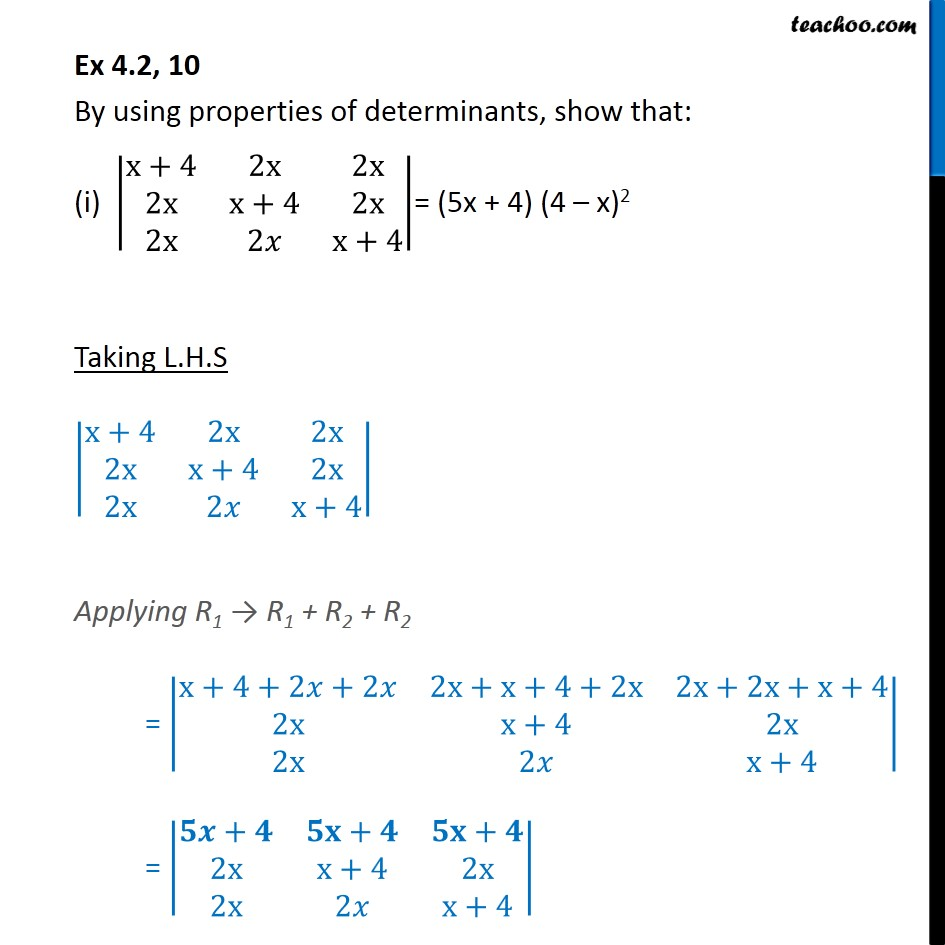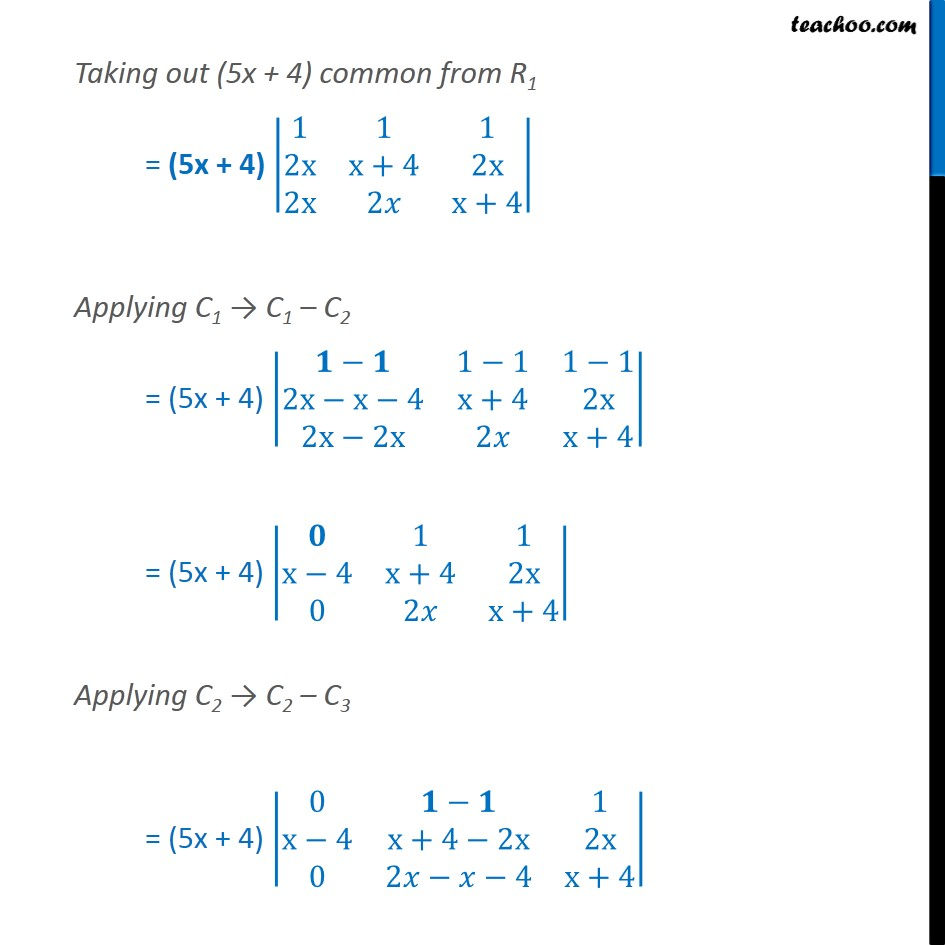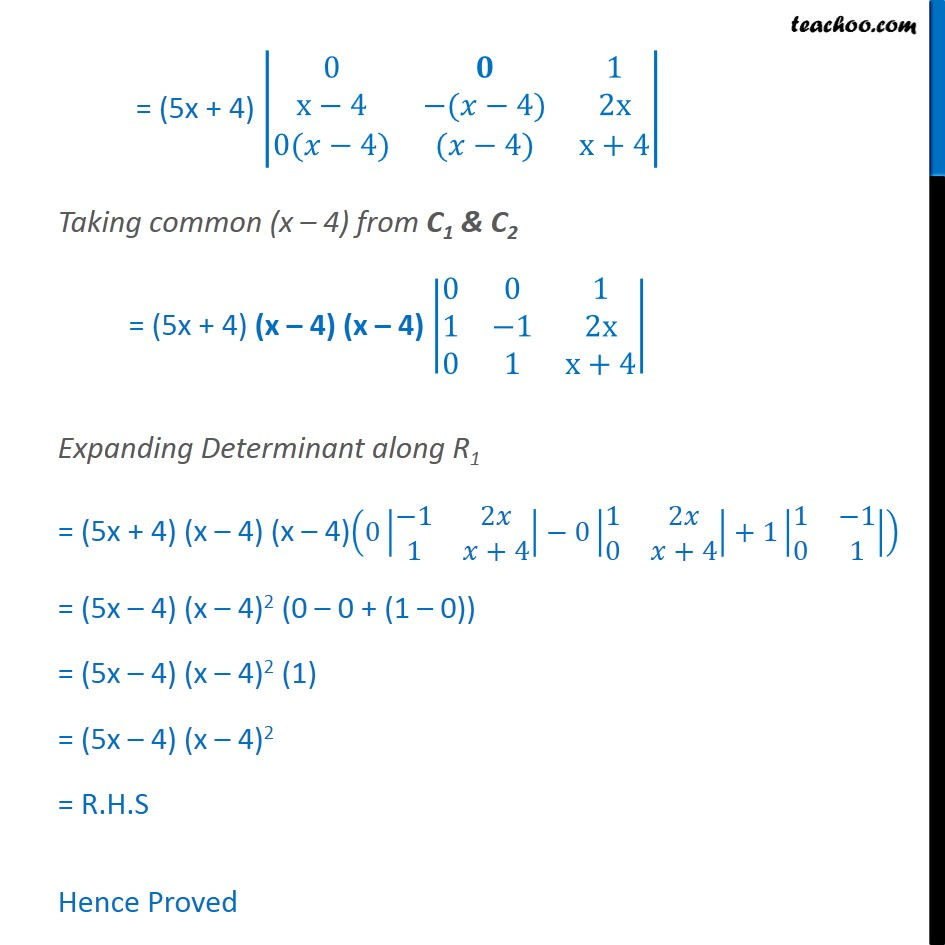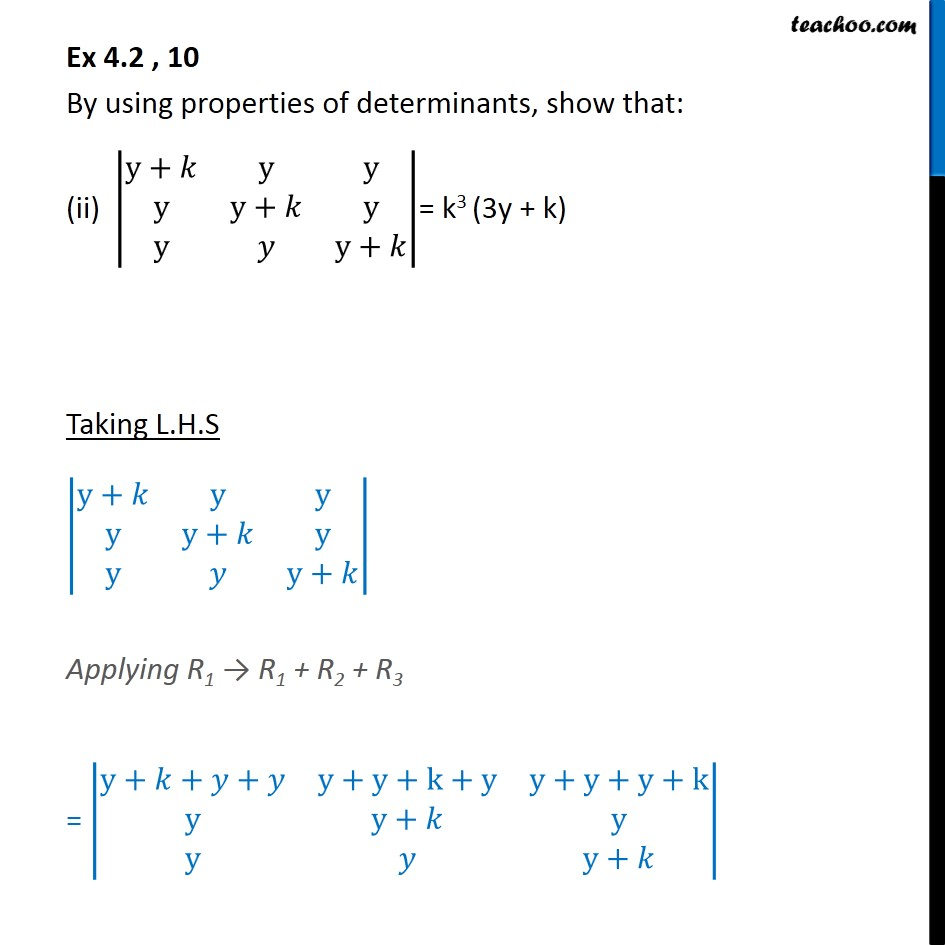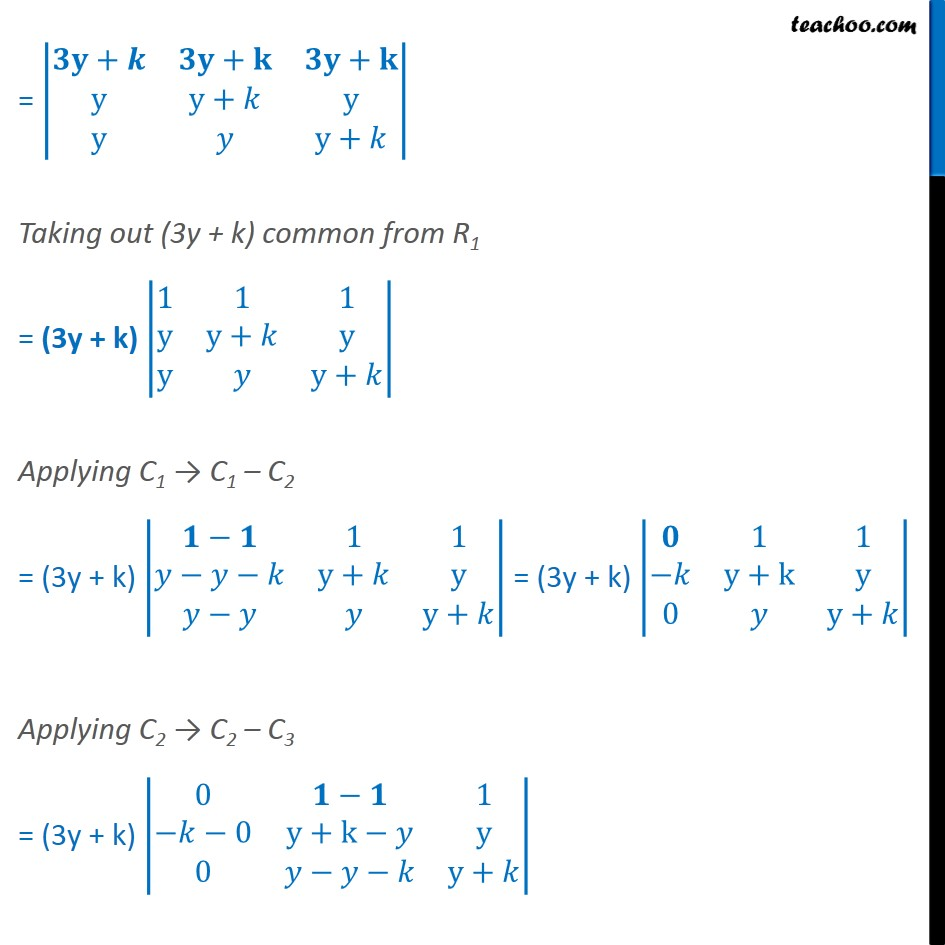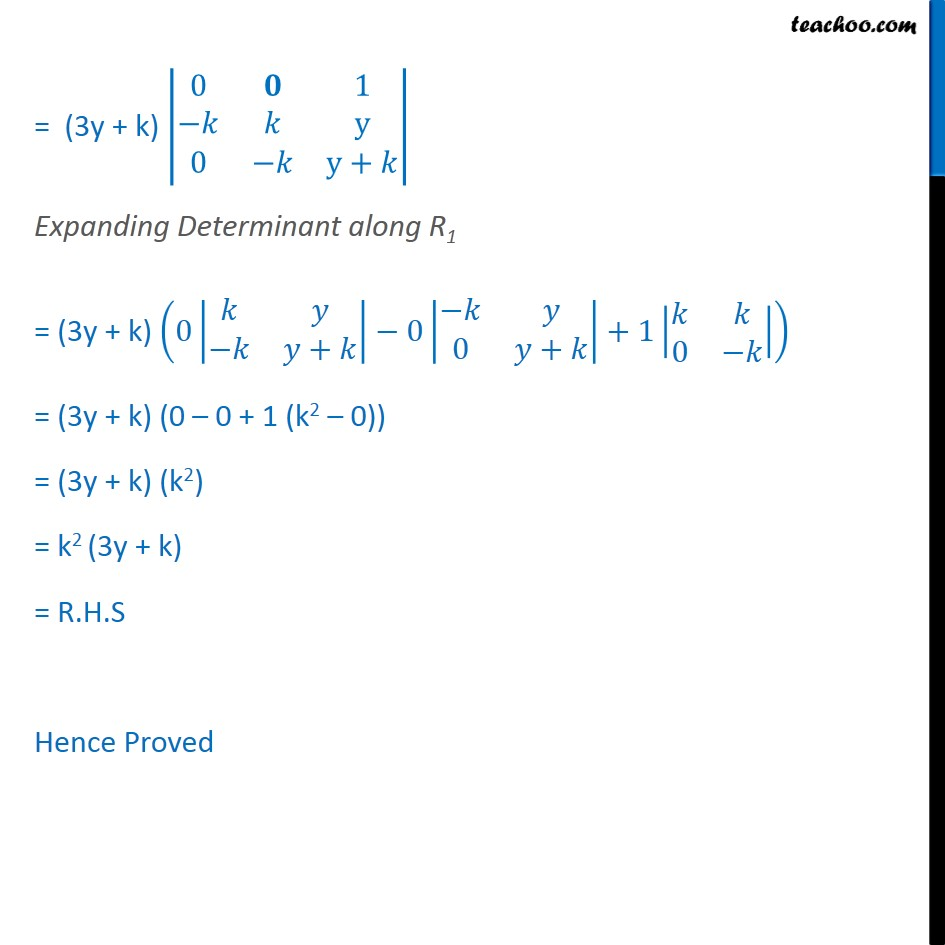Subscribe to our Youtube Channel - https://you.tube/teachoo

1. Chapter 4 Class 12 Determinants
2. Serial order wise
3. Ex 4.2

Transcript

Ex 4.2, 10 By using properties of determinants, show that: (i) x+4 2x 2x 2x x+4 2x 2x 2 x+4 = (5x + 4) (4 x)2 Taking L.H.S x+4 2x 2x 2x x+4 2x 2x 2 x+4 Applying R1 R1 + R2 + R2 = x+4+2 +2 2x+x+4+2x 2x+2x+x+4 2x x+4 2x 2x 2 x+4 = + + + 2x x+4 2x 2x 2 x+4 Taking out (5x + 4) common from R1 = (5x + 4) 1 1 1 2x x+4 2x 2x 2 x+4 Applying C1 C1 C2 = (5x + 4) 1 1 1 1 2x x 4 x+4 2x 2x 2x 2 x+4 = (5x + 4) 1 1 x 4 x+4 2x 0 2 x+4 Applying C2 C2 C3 = (5x + 4) 0 1 x 4 x+4 2x 2x 0 2 4 x+4 = (5x + 4) 0 1 x 4 ( 4) 2x 0( 4) ( 4) x+4 Taking common (x 4) from C1 & C2 = (5x + 4) (x 4) (x 4) 0 0 1 1 1 2x 0 1 x+4 Expanding Determinant along R1 = (5x + 4) (x 4) (x 4) 0 1 2 1 +4 0 1 2 0 +4 +1 1 1 0 1 = (5x 4) (x 4)2 (0 0 + (1 0)) = (5x 4) (x 4)2 (1) = (5x 4) (x 4)2 = R.H.S Hence Proved Ex 4.2 , 10 By using properties of determinants, show that: (ii) y+ y y y y+ y y y+ = k3 (3y + k) Taking L.H.S y+ y y y y+ y y y+ Applying R1 R1 + R2 + R3 = y+ + + y+y+k+y y+y+y+k y y+ y y y+ = + + + y y+ y y y+ Taking out (3y + k) common from R1 = (3y + k) 1 1 1 y y+ y y y+ Applying C1 C1 C2 = (3y + k) 1 1 y+ y y+ = (3y + k) 1 1 y+k y 0 y+ Applying C2 C2 C3 = (3y + k) 0 1 0 y+k y 0 y+ = (3y + k) 0 1 y 0 y+ Expanding Determinant along R1 = (3y + k) 0 + 0 0 + +1 0 = (3y + k) (0 0 + 1 (k2 0)) = (3y + k) (k2) = k2 (3y + k) = R.H.S Hence Proved

Ex 4.2